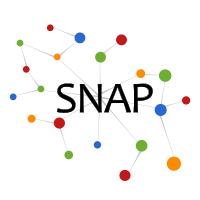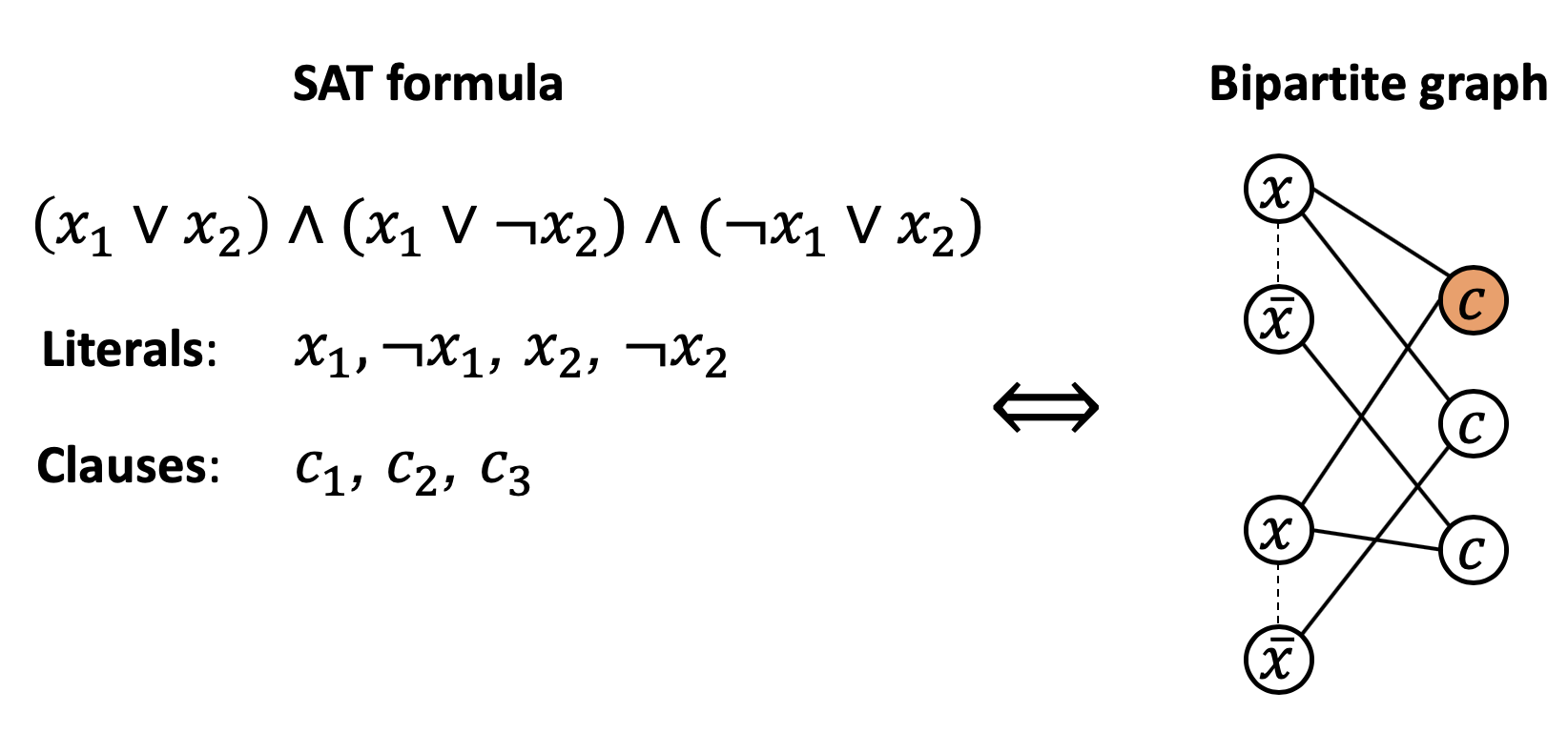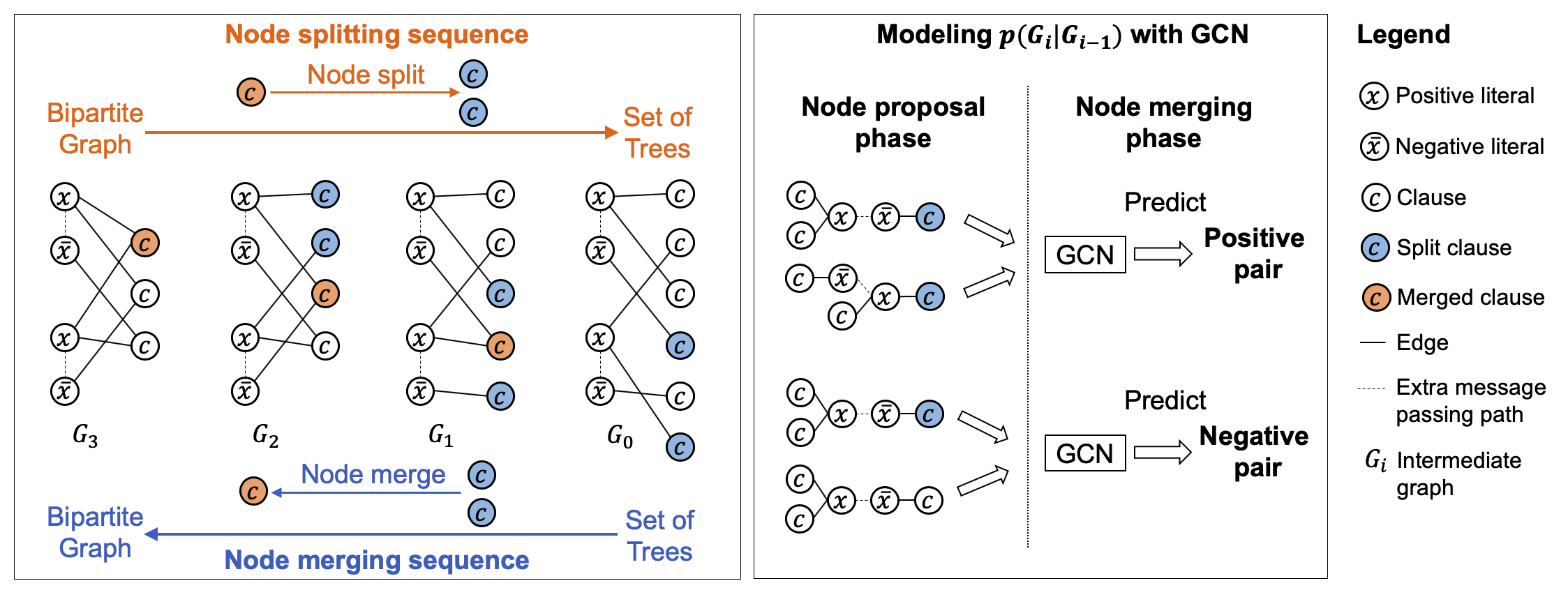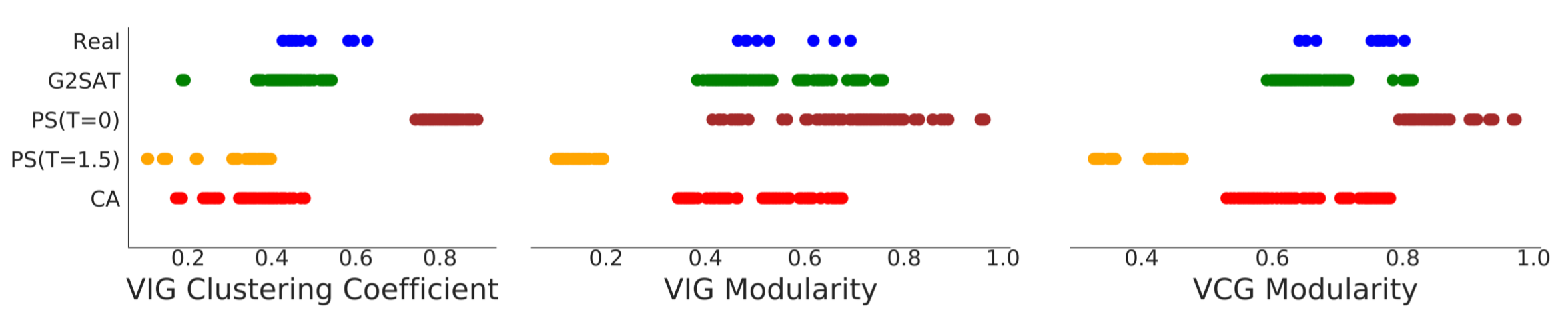# G2SAT: Learning to Generate SAT Formulas

G2SAT is the first deep generative framework that learns to generate SAT formulas from a given set of input formulas. We transformed SAT formulas into latent bipartite graph representations which are modeled using a specialized deep generative neural network.

## Motivation

The Boolean Satisfiability (SAT) problem is the canonical NP-complete problem and is fundamental to computer science. Developing and evaluating practical SAT solvers relies on extensive empirical testing on a set of real-world benchmark formulas. However, the availability of such real-world SAT formulas is limited. G2SAT can generate SAT formulas that closely resemble given real-world SAT instances, thus can help with this issue.

## Method

G2SAT is based on the observation that every SAT formula can be converted into a corresponding bipartite graph, via a bijective mapping. Here is an example.Instead of applying out-of-the-box graph generative models, e.g., GraphRNN, we designed a specialized bipartite graph generative model in G2SAT. Our key insight is that any bipartite graph can be generated by starting with a set of trees, and then applying a sequence of node merging operations over the nodes from one of the two partitions. As we merge nodes, trees are also merged, and complex bipartite structures begin to appear. To train G2SAT with such a process, we can generate training data via applying the reverse operation, node splitting, over a given bipartite graph. In this manner, a set of input bipartite graphs (SAT formulas) can be characterized by a distribution over the sequence of node merging operations. This process is illustrated by the below figure, left.Given this formulation, the way to generate a bipartite graph is quite simple, illustrated by the above figure, right. First, in node proposal phase, we randomly sample node pair candidates for node merging operations. Then, in node merging phase, we use a Graph Convolutional Neural Network (GCN) to predict which node pair is most likely to be merged.

We show that G2SAT is powerful in generating similar SAT graphs: it can closely fit all graph properties that we measure, and preserve relative SAT solver performance. The figure below shows the graph statistics range of training SAT formulas, and formulas generated by G2SAT and baseline methods.Please refer to our paper for detailed explanations and more results.

## Code

A reference implementation of G2SAT in Python is available on GitHub.

## Datasets

The datasets used by G2SAT are included in the code repository.

## Contributors

The following people contributed to G2SAT:
Jiaxuan You
Haoze Wu
Clark Barrett
Raghuram Ramanujan
Jure Leskovec

## References

G2SAT: Learning to Generate SAT Formulas. J. You*， H. Wu*, C. Barrett, R. Ramanujan, J. Leskovec. Neural Information Processing Systems (NeurIPS), 2019.